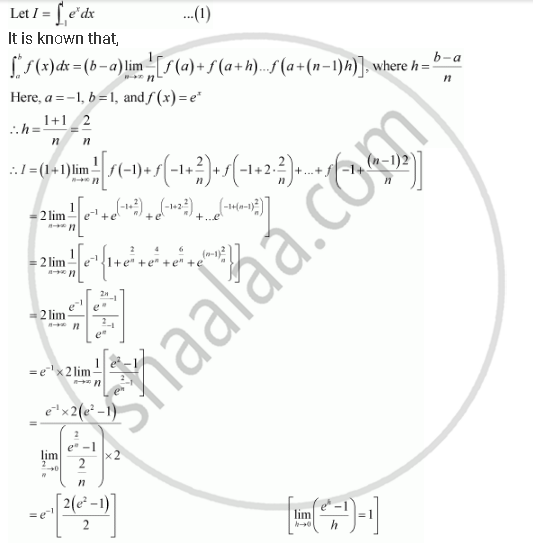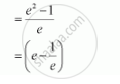Share

# Evaluate the Following Definite Integrals as Limit of Sums. Int_(-1)^1 E^X Dx - Mathematics

#### Question

Evaluate the following definite integrals as limit of sums int_(-1)^1 e^x dx

#### SolutionIs there an error in this question or solution?
Evaluate the Following Definite Integrals as Limit of Sums. Int_(-1)^1 E^X Dx Concept: Definite Integral as the Limit of a Sum.